﻿ The Electric Car Charging Strategy Based on the User's Intention and Its OptimizationPublications are Open
Access in this journal
Article Versions
Export Article
• Normal Style
• MLA Style
• APA Style
• Chicago Style
Research Article
Open Access Peer-reviewed

### The Electric Car Charging Strategy Based on the User's Intention and Its Optimization

Lu Mengtian
Journal of Automation and Control. 2017, 5(1), 16-19. DOI: 10.12691/automation-5-1-3
Published online: July 04, 2017

### Abstract

With the influence of electric vehicle technology development and environmental factors, more and more electric cars come into our life, we can expect large electric vehicles will connect to the grid in the future so it is necessary to study the influence of electric vehicle charging on distribution network system. This paper chooses New England 10 machine 39 bus system as an example to study the effect of electric vehicle charging on power grid loss in different permeability. The paper choose charging time and charging demand as constraint conditions, minimizing circuit loss rate as the objective function. The paper select the artificial fish swarm algorithm to optimize the simulation, through the Newton Raphson to calculate the power flow in MATLAB to get the contrasting curves of line loss rate before and after optimization, verify the effectiveness of the optimization model proposed in this paper.

### 1. Introduction

After entering twenty-first Century, the energy crisis appear, due to the deterioration of the environment and support of China's policy to promote the development of electric vehicle technology, electric vehicle has gradually penetrated into people's daily travel and the number is increasing day by day, it can be expected that in near future large-scale electric vehicles will be put into use. However, large-scale electric vehicles accessing to the grid to charge bring the new challenges to the operation and management of distribution network. If the users of electric vehicles disorderly charge, large-scale cluster charging behavior would adversely affect the stable and safe operation of power grid. Therefore, it is necessary to control the charging behavior of users reasonably and effectively.

Most of the related studies, mainly discusses the electric vehicle charging load and its optimization measures and minimization of charging cost, but only small part of studies discuss the voltage offset and harmonic problems; many research mainly discusses the effect of orderly charging model on the grid, but there is no specific details about the ordered charging strategy; several studies discuss some measures and take the user's intention into account but only optimized the charging load and did not consider the problems of network loss and voltage deviation of power distribution network.

On the basis of the large-scale cluster orderly charging, this paper considered users’ intention for controlling and meted users’ charging demand. The paper reduced charging times and fee as much as possible and considered the impact of electric vehicles charging on the network loss.

### 2. The Model of Electric Vehicles Charging Load and Its Calculation Method

2.1. The Model of Electric Vehicles Charging Load

In this paper we consider every user’s will for the regulation and control, so the regulation strategy needs to be built to meet the charge requirements. Users' different uses of electric vehicles lead to different behaviors of charge so the control strategy of the cluster also needs to consider the various user groups different charging requirements. The model is divided into three charging mode, respectively "night charge mode" and "daytime charge mode" and "immediate charge". The electric vehicle users should choose a charging mode for charging, if you choose nothing the latest choice of charging mode will be the default choice. The three charging modes are described as follows:

(1) "Night charge mode"

"Night charge mode" aimed at users charging at night. According to the traditional fuel vehicles travel rules, about ninety percent users belong to the "night charge mode" and the charging time is five p.m. to the second day seven a.m.. The charging mode will allow the users who selected this mode charge at the period of time mentioned above and the value of SOC smaller the charging priority the users get will be higher.

In the example simulation, the Rand function is used to generate a random number x between 0 and 1 and determine whether the x is in (0, 0.9), and use the night charging mode if x in this range.

(2) "Daytime charge model"

"Daytime charge mode" mainly for users charge at daytime. These users are not the mainstream, but can not be ignored. The main target of the mode is the users who needs to work at night whose charging time mainly at seven a.m. to five p.m.. The charging mode mainly arranges the users select the charging mode to charge during the charging period and the value of SOC smaller the charging priority the users get will be higher.

In the simulation, assuming that the number of "daytime charge mode" is 5% of the total number N, the Rand function generate a random number x between 0 and 1 and determine whether the x is in (0.9, 0.95) and use the daytime charge mode if x in this range.

(3) "Immediate charge"

The "immediate charge" is a fast charge model that takes into account the special charging requirements of some users. In charging demand simulation, the time limit of the model is 80 minutes.

In the simulation, assuming the "immediate charge" users accounted for N in 5%, Rand function is used to generate a random number x between 0 and 1 and determine whether the x is in (0.95, 1.0), and use the immediate charge mode if x in this range.

2.2. Charging Constraints

(1) In order to improve the number of users involved in charge allocation, the charging optimization model set the making the charging cost lowest as the optimization target: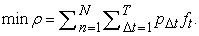(1)

In the formula,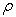is total cost of charging for all electric cars in the total period of charging time; N is the number of charging electric vehicles connected to the grid in total period of time; T is total time of 24 hours, which was divided into the 96 periods of time, each of the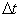is 15min;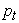is the charging power for electric cars under the period of time;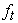is the price of every moment.

(2) All three charge modes have charging time constraint so the charge time constraint is as follows: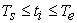(2)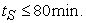(3)

In the formulas,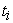is the charging time of ith electric vehicle in "night charge mode" and "daily charge" mode o,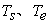respectively indicates the start-time and the end-time;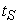is duration of "immediate charge mode".

(3) Charging demand constraint;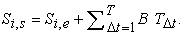(4)

In the formula, the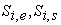indicate the SOC value of the ith electric vehicle before and after charging;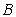represents the added SOC value at each time period.

(4) Objective function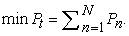(5)

In the formula,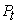represents the total power loss rate of the line,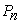represents the loss rate on the N branch, and the total branch is N. This paper takes the minimum total loss rate as the target.

2.3. Impact of Electric Vehicles Charging on the Loss Rate of Distribution Network

This paper uses Newton Raphson method to calculate the current distribution of cases. By dealing with the results of the calculation, we can obtain line load loss rate and no-load loss rate and total loss rate under different permeability, as shown in Figure 1, Figure 2, before the electric vehicles connected to the grid, due to a lower rate of load the loss rate is relatively low and the transformer no-load loss rate is higher, so the total loss rate is generally low, with the increase of permeability, the electric vehicle charging load increases, the load loss rate increased gradually, on the contrary, the transformer load loss rate decreased, but the total loss rate increased gradually.

• Figure 2. TOTAL loss rate
2.4. Fish Algorithm

The artificial fish swarm algorithm is a optimization method proposed by imitation of the fish behavior, which can solve nonlinear function optimization problems, it does not need to know about the specific information, only need to compare quality of question and has wide application scope and fast convergence speed.

Artificial fish algorithm steps as follows:

(1) set the parameter, including the individual number of artificial fish stocks N, the initial position of each artificial fish, the maximum step length of artificial fish movement, the visual field of artificial fish, the number of times to retry and the crowding factor.

(2) To calculate the fitness value of each artificial fish, record the status of the global optimum artificial fish, and record the data in the bulletin board.

(3) To evaluate each artificial fish and analyze its foraging behavior, clustering behavior, rear end collision behavior and random behavior.

(4) To perform artificial fish selection, updating the location information of each artificial fish.

(5) To update the status of global optimum artificial fish.

(6) To output the result if the end condition of loop is satisfied otherwise jump to step (2).

### 3. Example Simulation

3.1. Network Model

In this paper, New England 10 machine 39 node standard system is taken as an example to study the impact of electric vehicle charging on the network loss. The connection is shown in Figure 3. The system has 39 nodes, 12 transformers and 34 lines. The reference voltage of the system is 345KV and three-phase power reference value is 10MVA and the power factor is 0.95. Joints 1 through 29 are PQ nodes and the 31th node is the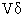node and the rest of nodes are PV nodes. The paper assumes that the per-unit value is 1.

• Figure 3. Topology of New England39 node distribution system network
3.2. Parameter Setting and Selection of State of Fish Swarm Algorithm

1. Parameter settings

The perceived distance Visual of artificial=5.0 male fish, the largest mobile Step=0.3 mobile step of artificial fish, artificial fish number N=30, the maximum number of iterations of Try-number=50

2. Status selection

(1) the selection of foraging behavior: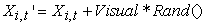(6)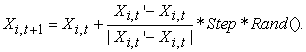(7)

As shown in (6), at t time the ith artificial fish is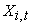and a state namely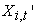randomly is generated in the induction region. Rand function generates a random number between 0 and 1 and calculate the maximum according to the random state determined by type (6). If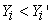, to walk one step towards the direction, otherwise choose a new state to judge until it meets the conditions. When the number of attempts is larger than Try-number, to walk one step randomly if the type (7) is not met.

(2) The choice of swarm behavior: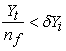(8)is the state of the ith artificial fish at the t moment.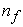is the number of artificial fish in the field of vision. When the in-equation (8) is met, it indicates that the centers of other artificial fish have more food and are not crowded, so we can put the center position namely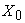into the formula (7), indicating a step forward to direction of the artificial fish.

(3) The choice of rear-end collision:

The rear-end collision is a behavior that moving to the near optimal artificial fish.is the state of the ith fish at t moment. If the artificial fish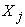of which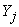is the maximum value of the artificial fish in the field satisfies inequality type(8), indicating that the artificial fishhas high food concentration and not crowded, we will pluginto formula (7) which represents a step forward toward.

(4) The choice of random behavior:

The random behavior represents the search for an optimal value in a larger range, indicating moving toward the overall which is randomly chosen in the field of view.

3.3. MATLAB Simulation

According to the known data, the paper calculated power flow of New England 10 machine 39 bus system by Newton Raphson method in MATLAB so we obtained the admittance matrix of the New England 39 system. Secondly, the paper generated the Jacobi matrix to get the correction and correction equation and then iterating until convergence criterion is satisfied so the power flow calculation the ended.

• Figure 4. Comparison of total loss rate before and after optimization

Figure 4 shows the optimization results. By regulating the power of electric vehicle charging under the artificial fish swarm optimization model, the paper obtained the total loss rates of New England 39 system before and after optimization in different permeability. As shown in Figure 4, the maximum value of total loss rate of line before and after optimization respectively is 7.8% and 6.23% and we can find that the optimized value is 20.1% lower than that before optimization. After optimization, as the permeability increases gradually, the total loss of the circuit is reduced first and then increased and reached the lowest value in the penetration rate of 30%, 1.6% less than the value before optimization. In summary, the optimization effect is ideal.

### 4. Summary

This paper presents a charging model of electric vehicle based on user's intention and the charging behavior is divided into three modules respectively are solved. The model considers the constraints of charging time and charging demand, with minimizing total line loss rate as the objective function, using New England 10 machine 39 node for example simulation. By using the Newton Raphson algorithm for power flow calculation, the paper obtained the no-load loss rate, the line load loss rate and total loss rate under different permeability. The model was optimized by artificial fish swarm algorithm and obtained comparison of total loss rate before and after optimization under different permeability and the results verified the validity of the optimization model.

### Acknowledgements

This work was conducted partly at Nanjing normal university Research Lab.

### References

  HADLEY S W ，TsVETK0VA A. Potential impacts of plug—in hybrid electric vehicles on regional power generation. Knoxville, TN, USA: ORNL, 2008. In article View Article  CHEN Li-dan, ZHANG Yao. Study on the influence of electric vehicles random charging on distribution network. Journal of electric power science and technology, 2016, 31(1). In article  DANG Jie, TANG Yi, NING Jia, FU Xiangyun, A strategy for distribution of electric vehicles charging load based on user intention and trip rule. Power System Protection and Control, 2015, 43(16). In article  ZHAN Kaiqiao，SONG Yonghua，HU Zechun，XU Zhiwei，JIA Long, Coordination of Electric Vehicle Charging to Minimize Active Power Losses. Proceedings of the CSEE, 2012, 32(31). In articleThis work is licensed under a Creative Commons Attribution 4.0 International License. To view a copy of this license, visit http://creativecommons.org/licenses/by/4.0/